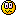# Sorry your browser is not supported!

You are using an outdated browser that does not support modern web technologies, in order to use this site please update to a new browser.

Browsers supported include Chrome, FireFox, Safari, Opera, Internet Explorer 10+ or Microsoft Edge.

### DarkBASIC Discussion / skydiving

Message
Posted: 4th Mar 2013 17:25

This is what i have so far. I need help making it a top view and getting the object to move downward.
Thanks,
Nathan

You are entering the Wu Dynasty. Boomshakalaka
-Nathan
Posted: 4th Mar 2013 22:33
Well moving downward is easy, it's just a matter of calculating a new position and then using the POSITION OBJECT command to reposition the box.

The calculating is a bit more difficult (unless you go for the easy EASY way of a constant falling speed, in which case, skip this next part, but you should read, because physics and math are fun)

The simple equation set is:

acceleration=constant
velocity=acceleration*time+initial_velocity
position=.5*acceleration*time^2+initial_velocity*time+initial_position

However, these will ONLY give you basic motion, ie, your skydiver will speed up to infinity the longer he is in the air. We know that this ISN'T how life is, as there is a "terminal velocity", that is, a speed at which the wind resistance negates gravity and your fall speed stops increasing. Effectively: acceleration is no longer constant.

So let's try this:

The terminal velocity of a person is roughly 195 km/h (122 mph or 54 m/s) according to wikipedia. Lets set the acceleration to something dealing with that:

acceleration=(terminal_velocity-velocity)/terminal_velocity

So what is that? That is a modified percent change equation. We are essentially evaluating how far away from the terminal velocity we are and then converting that into a percentage. This would need to be multiplied by a value to make this percent MEAN something. So let's multiply it by the acceleration due to gravity (normally 9.8 m/s^2 or 32 ft/s^2 for those of us in the States).

acceleration=gravity*(terminal_velocity-velocity)/terminal_velocity

Then integrating* 2 times:

velocity=acceleration*time+initial_velocity
position=0.5*acceleration*time^2+initial_velocity*time+initial_position

And that's the math.
*Calculus aside:*
I put an * by "integrating" because we are taking some shortcuts here. This is an iterative approach, not one that is good for pencil and paper. Why? Because our acceleration equation uses Velocity, which should be a function of time and, thus, have an integration that is less nice. We are assuming that the current velocity is, for all intents and purposes, constant, we then find the NEW velocity through the integrations.
*End of Calculus aside*

I made up the acceleration formula, it isn't accurate to physics, but it is simple and should work fine with what you are doing (accurate stuff will require the mass of the object, the cross sectional area, the drag coefficient, etc which we don't want to deal with).

So how do you apply any of this? Time is more of an "us" thing rather than a "computer thing" so lets do the easy thing with our units: instead of "seconds", lets use "code cycles". That way, every time the math is run, the time has only increased by 1, which lets us skip a lot of the less kind math. Also, all of those references to time require a falling timer, so lets get rid of those by tweeking the dependency on initial settings. So every time we loop through our code, it will look something like this:

You will need to fine tune your gravity and your terminal_velocity constants in order to make the thing not suddenly EXPLODE with speed (keep in mind, your code loops many times a second, and this will increase your speed by 54 every loop, so, yeah).

----------------STOP SKIPPING HERE!--------------------

Now if you skipped everything, because you don't want a lot of math involved, and just want to fall at a constant speed, you would just use the last one with 0 acceleration, basically:

position#=position#+fallSpeed#

Which, while easier, isn't as smooth as the longer method.

Ultimately, however, you won't be able to tell anything is happening, because all you have is a box in a blue world. It could fall but you wouldn't see anything going by, so you wouldn't be able to tell (you have no frame of reference to determine motion by). So you'll need clouds, birds, and maybe a plane or two to fly by so that you can really feel like your falling, instead of just existing.

And for your camera question:

POSITION CAMERA Object Position X(ObjectNumber),Object Position Y(Object Number)+distance#,Object Position Z(Object Position)
POINT CAMERA Object Position X(ObjectNumber),Object Position Y(Object Number), Object Position Z(ObjectNumber)

Shortcuts include:
Your camera probably won't change orientation at all, so you can position it and point it once, then all you have to do is position it(because you will always be above the player looking straight down).

There is also the SET CAMERA TO FOLLOW command, but I always have trouble getting that to work the way I want it to.

Hope this helps a bit, let me know if you have any questions!

For the record, I really like math based solutions, so that is why all of that ^ is there. There might be another solution that doesn't involve as much math, but where's the fun in that?

Great Quote:
"Time...LINE??? Time isn't made out of lines...it is made out of circles. That is why clocks are round!" -Caboose
Posted: 5th Mar 2013 17:42
So much code man...